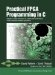# Section 10.1. Rethinking an Algorithm for Performance

### 10.1. Rethinking an Algorithm for Performance

Chapter 9 presented an example of data encryption using the triple-DES encryption algorithm, using a legacy C implementation of the algorithm as a basis for the application. As indicated in that chapter, the purpose of the example was to demonstrate how to prototype a hardware implementation in an FPGA, using C code that had previously been written for and compiled to an embedded processor. When hardware simulation of the resulting RTL was performed, it was determined that the rate at which the algorithm could generate encrypted blocks of data was approximately one block approximately every 191 cycles. This rate is faster in terms of clock cycles than what could be achieved in a software implementation (using an embedded processor for comparison), but given the much higher clock rates possible in modern processors, this is unlikely to be a satisfactory result for FPGA-based hardware acceleration.

To increase the performance of this application, we begin by taking a closer look at the algorithm itself and how it was initially implemented as an Impulse C process. To simplify the example for the purpose of discussion, we will focus on only one of the three single-DES passes of this algorithm. The following are excerpts from the original legacy C code that processes one 64-bit block of eight characters:

` left = ((unsigned long)block << 24)     | ((unsigned long)block << 16)     | ((unsigned long)block << 8)     | (unsigned long)block;    right = ((unsigned long)block << 24)     | ((unsigned long)block << 16)     | ((unsigned long)block << 8)     | (unsigned long)block;    work = ((left >> 4) ^ right) & 0x0f0f0f0f;    right ^= work;    left ^= work << 4;    work = ((left >> 16) ^ right) & 0xffff;    right ^= work;    left ^= work << 16;    work = ((right >> 2) ^ left) & 0x33333333;    left ^= work;    right ^= (work << 2);    work = ((right >> 8) ^ left) & 0xff00ff;    left ^= work;    right ^= (work << 8);    right = (right << 1) | (right >> 31);    work = (left ^ right) & 0xaaaaaaaa;    left ^= work;     right ^= work;    left = (left << 1) | (left >> 31); `

The preceding block prepares the data by arranging the 64-bit block into two 32-bit values and performing some initial permutations on the data. Simple bit-level manipulations such as this will be easily converted by the Impulse C compiler into hardware logic requiring only a single cycle. Reading the data from the block array, however, will require at least eight cycles because only one value per cycle can be read from memory. Reducing the number of accesses required to memory is one key optimization that we will consider for this algorithm.

Next, consider the following excerpts, in which a relatively complex macro has been defined as part of the calculation:

` #define F(l,r,key){\        work = ((r >> 4) | (r << 28)) ^ key;\        l ^= Spbox[work & 0x3f];\        l ^= Spbox[(work >> 8) & 0x3f];\        l ^= Spbox[(work >> 16) & 0x3f];\        l ^= Spbox[(work >> 24) & 0x3f];\        work = r ^ key;\        l ^= Spbox[work & 0x3f];\        l ^= Spbox[(work >> 8) & 0x3f];\        l ^= Spbox[(work >> 16) & 0x3f];\        l ^= Spbox[(work >> 24) & 0x3f];\  } `

This macro is subsequently used in the following section of the algorithm:

` F(left,right,Ks); F(right,left,Ks); F(left,right,Ks); F(right,left,Ks); F(left,right,Ks); F(right,left,Ks); F(left,right,Ks); F(right,left,Ks); F(left,right,Ks); F(right,left,Ks); F(left,right,Ks); F(right,left,Ks); F(left,right,Ks); F(right,left,Ks); F(left,right,Ks); F(right,left,Ks); `

This code performs the main operation of the encryption/decryption. Some simple bit manipulations are performed, but there are also many references to both one and two-dimensional array elements. Each instantiation of the macro F performs eight loads from the Spbox array, and F itself is instantiated 16 times for a total of 128 loads. From that you can easily see that this block of code will require at least 128 cycles.

The final block of code performs some final permutations on the two 32-bit values and arranges the result in the output array:

` right = (right << 31) | (right >> 1); work = (left ^ right) & 0xaaaaaaaa; left ^= work; right ^= work; left = (left >> 1) | (left  << 31); work = ((left >> 8) ^ right) & 0xff00ff; right ^= work; left ^= work << 8; work = ((left >> 2) ^ right) & 0x33333333; right ^= work; left ^= work << 2; work = ((right >> 16) ^ left) & 0xffff; left ^= work; right ^= work << 16; work = ((right >> 4) ^ left) & 0x0f0f0f0f; left ^= work; right ^= work << 4; block = (unsigned char) (right >> 24); block = (unsigned char) (right >> 16); block = (unsigned char) (right >> 8); block = (unsigned char) right; block = (unsigned char) (left >> 24); block = (unsigned char) (left >> 16); block = (unsigned char) (left >> 8); block = (unsigned char) left; `

Again, the simple bit manipulations being shown here will be easily compiled into a single-cycle operation. Storing the data into the block array will require at least eight cycles, however, because only one data value can be written to memory per cycle.

Using this original, unmodified code, the CoDeveloper tools generate a hardware implementation that uses close to 3,000 slices in a Xilinx Virtex-II FPGA and perform just 10.6 times faster than an equivalent algorithm running in software on an embedded MicroBlaze processor. In the following sections we will demonstrate a number of refinements that can be applied in this example to obtain better results, both in terms of clock cycles and in the size of the resulting logic.Practical FPGA Programming in C
ISBN: 0131543180
EAN: 2147483647
Year: 2005
Pages: 208

Similar book on Amazon